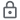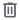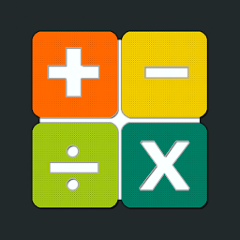# Математика: устный счет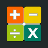5K+Everyone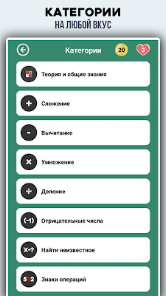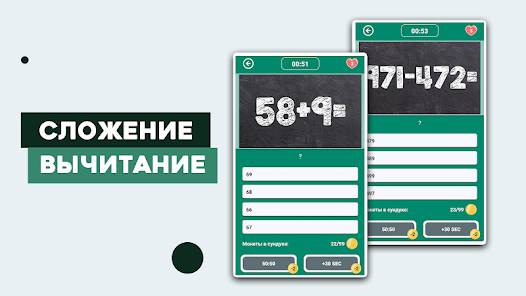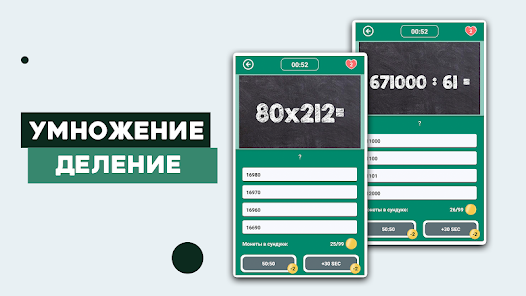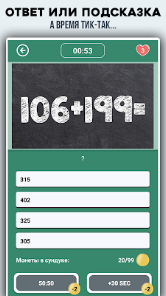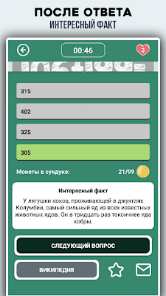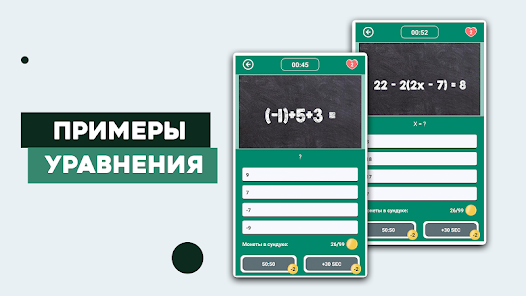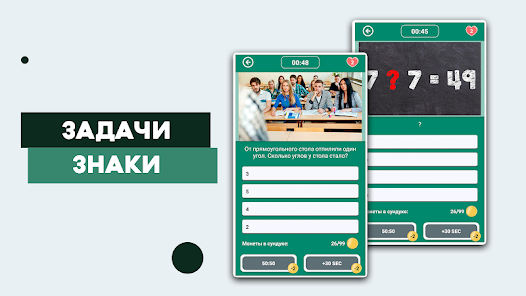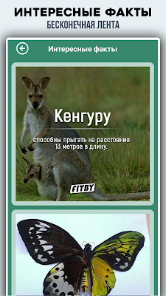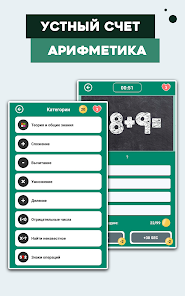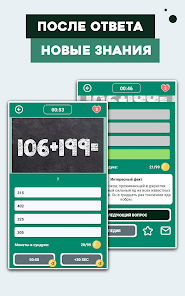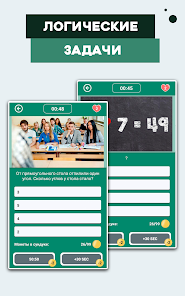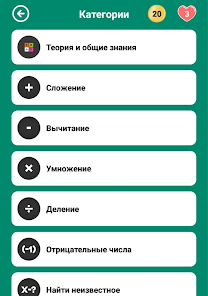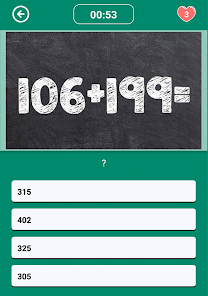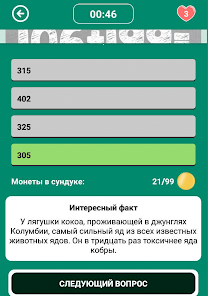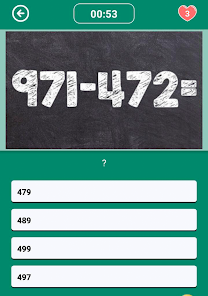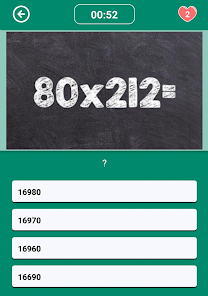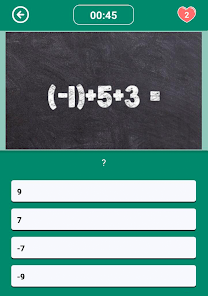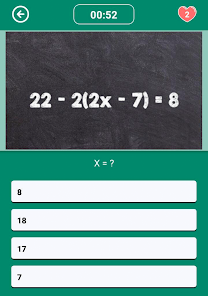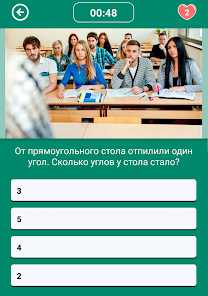Install the quiz Math: Counting, Quizzes, Arithmetic is an entertaining game that will be useful for those people who want to improve their math skills and increase their brain limits.

Math: Maths, Quizzes, Arithmetic - The Maths Quiz will help you gain important math skills.
Learn and train mental arithmetic, addition, subtraction, multiplication table, division, use our math examples and equations.
Before you notice, all the mathematical tricks will be mastered and learned!

For a more interesting quiz, after each answer you will receive an interesting fact about the world!

🎓 STUDY
More than 1000 examples of mental arithmetic in math:
☆ theory and test of general knowledge in mathematics
☆ addition and subtraction of single digits
☆ addition and subtraction of double digits, multiplication of single digits (multiplication table)
☆ addition and subtraction of three-digit, multiplication and division of two-digit by single
☆ multiplication and division of two-digit and three-digit
☆ negative numbers
☆ equations and find the unknown
☆ percent math problems
☆ oral counting will be useful in preparing for exams
☆ mental arithmetic is the best brain trainer
☆ elementary mathematics
☆ logic puzzles

🏁 USEFUL
☆ Count in your mind and activate your brain using the app.
☆ Test your skills in addition, subtraction, division, multiplication and mixed mode.
☆ This is a good quiz game to improve your mental arithmetic and good for brain training.
☆ Read interesting facts while playing, gain new knowledge!

🎮 HOW TO PLAY:
☆ Choose a theme that interests you!
☆ Get points and coins for every question answered correctly!
☆ Coins give you the opportunity to use hints when passing the quiz!
☆ There are 2 clues at the bottom of each question that can increase your chances of getting the right answer

Mathematics: verbal counting, tests, arithmetic is very popular as an excellent memory training, attention training, intelligence training. Verbal counting is the most accessible mathematics that does not require any prior knowledge and skills.
Our math tests will help you remember everything you have forgotten a long time ago.
Updated on
Aug 10, 2022

## Data safety

Safety starts with understanding how developers collect and share your data. Data privacy and security practices may vary based on your use, region, and age. The developer provided this information and may update it over time.This app may share these data types with third parties
Device or other IDsNo data collected